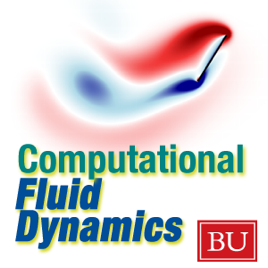21 episodes

There are many situations in which a computational approach is the ideal one for studying a problem. For example, in studying the flow of air in the breathing tract of a human, one can hardly do experiments! And even if we could experiment, how could we *see* what is happening? By simulation, we can both perform virtual experiments of the flow of fluids, and visualize the results in a myriad ways. Computational Fluid Dynamics (CFD) is vital for many applications of science and engineering. This course is designed for graduate students or advanced undergraduates.

Professor Lorena A Barba
Mechanical Engineering Department
Boston University# Computational Fluid Dynamics - ENG ME702 - Video Boston University

• Technology

There are many situations in which a computational approach is the ideal one for studying a problem. For example, in studying the flow of air in the breathing tract of a human, one can hardly do experiments! And even if we could experiment, how could we *see* what is happening? By simulation, we can both perform virtual experiments of the flow of fluids, and visualize the results in a myriad ways. Computational Fluid Dynamics (CFD) is vital for many applications of science and engineering. This course is designed for graduate students or advanced undergraduates.

Professor Lorena A Barba
Mechanical Engineering Department
Boston University

• video
Welcome to the course for iTunes subscribers

## Welcome to the course for iTunes subscribers

• 15 min
• video
Lecture 1: Introduction to Computational Fluid Dynamics.

## Lecture 1: Introduction to Computational Fluid Dynamics.

• 57 min
• video
Lecture 2: finite differences, model equations, and assignment steps 1 to 4

## Lecture 2: finite differences, model equations, and assignment steps 1 to 4

• 1 hr 9 min
• video
Lecture 3: FD explicit/implicit methods; Crank-Nicholson method; assignment steps 5 to 8

## Lecture 3: FD explicit/implicit methods; Crank-Nicholson method; assignment steps 5 to 8

• 1 hr 9 min
• video
Lecture 4: Analysis of numerical schemes; consistency, stability, convergence.

## Lecture 4: Analysis of numerical schemes; consistency, stability, convergence.

• 57 min
• video
Lecture 5: Analysis of numerical schemes; modified differential equation. Von Neumann stability analysis. Examples.

## Lecture 5: Analysis of numerical schemes; modified differential equation. Von Neumann stability analysis. Examples.

• 1 hr 25 min

## Customer Reviews

4.6 out of 5
21 Ratings

21 Ratings

RigelFive ,

### CFD - BU ME702 Lecture Series

I feel that this was a great set of lectures for an introduction to CFD. The style of writing out equations has been lost with the era of PowerPoint charts. To see the equations and discretizations being derived slowly and methodically I feel was a great help.

I wish we could see more of the simulations using the Enthought Python tool from the assignments.... but that would make things too easy on the student.

After this class, I am now reading my Tannehill, Anderson and Pletcher textbook with much better understanding.